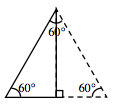Home > A2C > Chapter 8 > Lesson 8.1.2 > Problem8-15

8-15.
1. Copy the triangles below. Homework Help ✎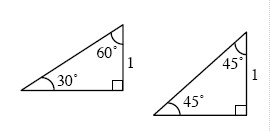1. Label the missing sides with their exact lengths. That is, leave your answers in radical form.

2. The 30° − 60° − 90° triangle is sometimes called a half-equilateral. Draw a picture to illustrate this, and explain how that fact can be used to help label the missing sides in part (a).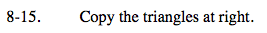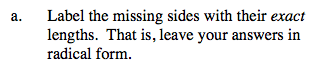Refer to the Math Notes box for this lesson.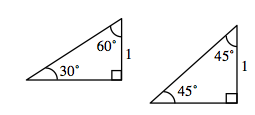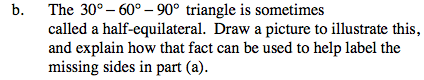Reflect the 30°-60°-90° triangle across the long leg. This will help you find the hypotenuse.
Use the Pythagorean Theorem to find the length of the long leg.